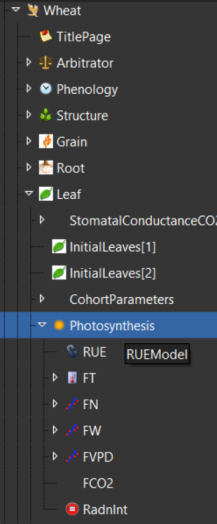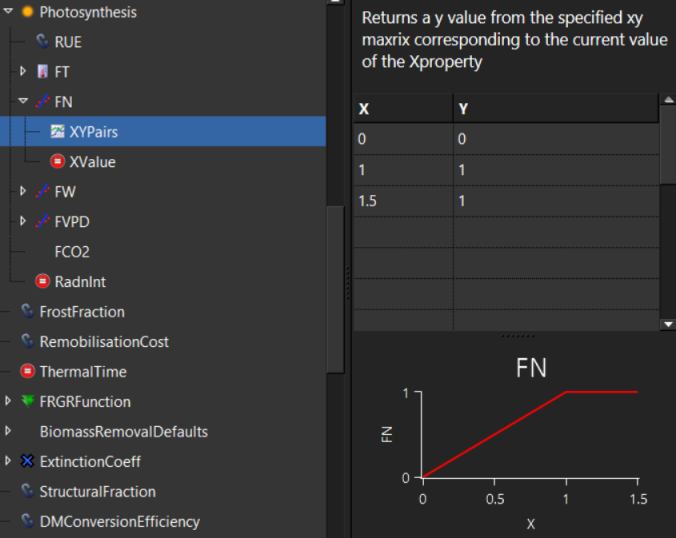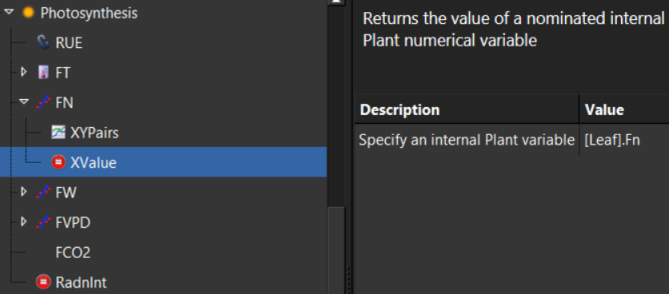# How PMF functions work

In this tutorial we will explain how Plant Modelling Framework (PMF) functions work. We will use the wheat leaf photosynthesis model as an example.

Prerequisite: It is suggested you read how to build a model first.

# 1. The PMF leaf organ

There are multiple PMF leaf organs that different crops use. For this tutorial we will examine the organ Leaf in Leaf.cs. This is the organ that wheat uses. The other leaf organs work the same way with respect to photosynthesis so the information in this tutorial is relevant for other crop models in APSIM.

``````/// <summary>The photosynthesis</summary>
IFunction Photosynthesis = null;
``````

The leaf organ has a link to a photosynthesis model that is of type IFunction. Leaf has a single call to this Photosynthesis function to get the amount of dry matter fixed for the day (g/m2):

`````` DMSupply.Fixation = Photosynthesis.Value();
``````

The leaf organ knows nothing of the implementation of Photosynthesis other than it is an IFunction.

# 2. IFunction

As we saw in the previous code block, the Photosynthesis IFunction has a Value method that returns a double. All PMF functions (models) implement IFunction and so must supply an implementation of this method.

``````public interface IFunction
{
/// <summary>Gets the value of the function.</summary>
double Value(int arrayIndex = -1);
}
``````

# 3. Where is the implementation of photosynthesis?

Most crop models in APSIM use the same implementation of photosynthesis but parameterise it in different ways. The flexibility exists though for the model developer to use a different implementation.

To determine what implementation and parameterisation are used for a particular crop model:

• From the user interface, open a wheat example.
• By default the wheat model won’t show it’s structure or parameterisation. To show more detail, right click on the wheat model and select ‘Show model structure’.The image above shows Photosynthesis selected (under wheat) and the tooltip showing RUEModel. This tells us the c# class being used is RUEModel. The image also shows us that there are 7 child models of RUEModel, RUE, FT, FN, FW, FVPD, FCO2 and RandInt.

The user interface wheat visualisation comes from the wheat.json file in the resources, which is another way of determining what functions (models) wheat photosynthesis is using.

# 4. RUEModel

The source code of the RUEModel looks like this:

``````    public class RUEModel : Model, IFunction
{
/// <summary>The RUE function</summary>
IFunction RUE = null;

/// <summary>The FCO2 function</summary>
IFunction FCO2 = null;

/// <summary>The FN function</summary>
IFunction FN = null;

/// <summary>The FT function</summary>
public IFunction FT = null;

/// <summary>The FW function</summary>
IFunction FW = null;

/// <summary>The FVPD function</summary>
public IFunction FVPD = null;

/// <summary>Total plant "actual" radiation use efficiency.</summary>
[Units("gDM/MJ")]
public double RueAct
{
get
{
double RueReductionFactor = Math.Min(FT.Value(), Math.Min(FN.Value(), FVPD.Value()))
* FW.Value() * FCO2.Value();
return RUE.Value() * RueReductionFactor;
}
}

/// <summary>Daily growth increment of total plant biomass</summary>
/// <returns>g dry matter/m2 soil/day</returns>
public double Value(int arrayIndex = -1)
{
throw new Exception("NaN Radiation interception value supplied to RUE model");
throw new Exception("Negative Radiation interception value supplied to RUE model");
}
}
``````
• The RUEModel model implements IFunction.
• There are links to the seven functions that we noted where under photosynthesis in the user interface.
• There is a public property (output) called RueAct.
• Returns actual RUE by multiplying the smallest child function (FT, FN, FVPD) by FW and FCO2 and then RUE.
• The Value method provides the photosynthesis implementation.
• It calls the Value method of the RadnInt function to get the amount of radiation interception.
• It throws an exception if the radiationInterception is NaN or negative.
• It returns radiationInterception * RueAct.
• The level of indirection caused by having child functions for each of several multipliers offers great flexibility to the model developer in defining photosynthesis. It lets the model developer, from the user interface, change the individual multipliers from constants to a more complex linear interpolation or any other function type. For example: FT is a WeightedTemperatureFunction and FN is a LinearInterpolationFunction:The above image shows the visualisation of the FN linear interpolation. To determine what the X variable is you need to click on XValue in the simulation tree:The image above shows the model developer has specified [Leaf].Fn which means the FN linear interpolation will call the *Fn*property in Leaf to get the x value for the linear interpolation. The Fn property in leaf looks like this:

``````        [Units("0-1")]
public double Fn
{
get
{
double f;
double functionalNConc = (CohortParameters.CriticalNConc.Value()
- CohortParameters.MinimumNConc.Value() * CohortParameters.StructuralFraction.Value())
* (1 / (1 - CohortParameters.StructuralFraction.Value()));
if (functionalNConc <= 0)
f = 1;
else
f = Math.Max(0.0, Math.Min(Live.MetabolicNConc / functionalNConc, 1.0));

return f;
}
}
``````

The implementation of Fn then calls other functions: CriticalNConc, MinimumNConc and StructuralFraction which are all defined under Leaf.

# 5. Conclusion

This level of indirection (where one function calls another function which calls somewhere else) makes it very difficult to follow the logic of how photosynthesis works. The advantage though is that it is very flexible for the model developer to create models visually using the user interface. To help understand the PMF structure, it is recommended that you run the APSIM user interface showing the model structure beside the source code.